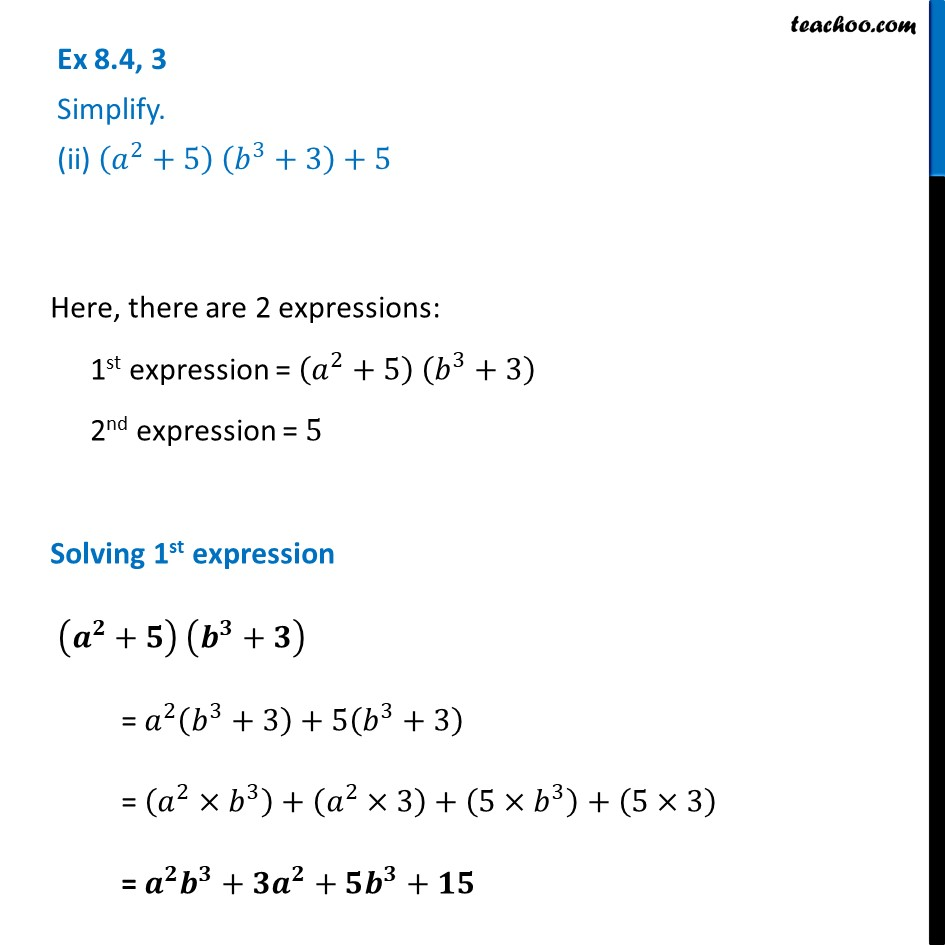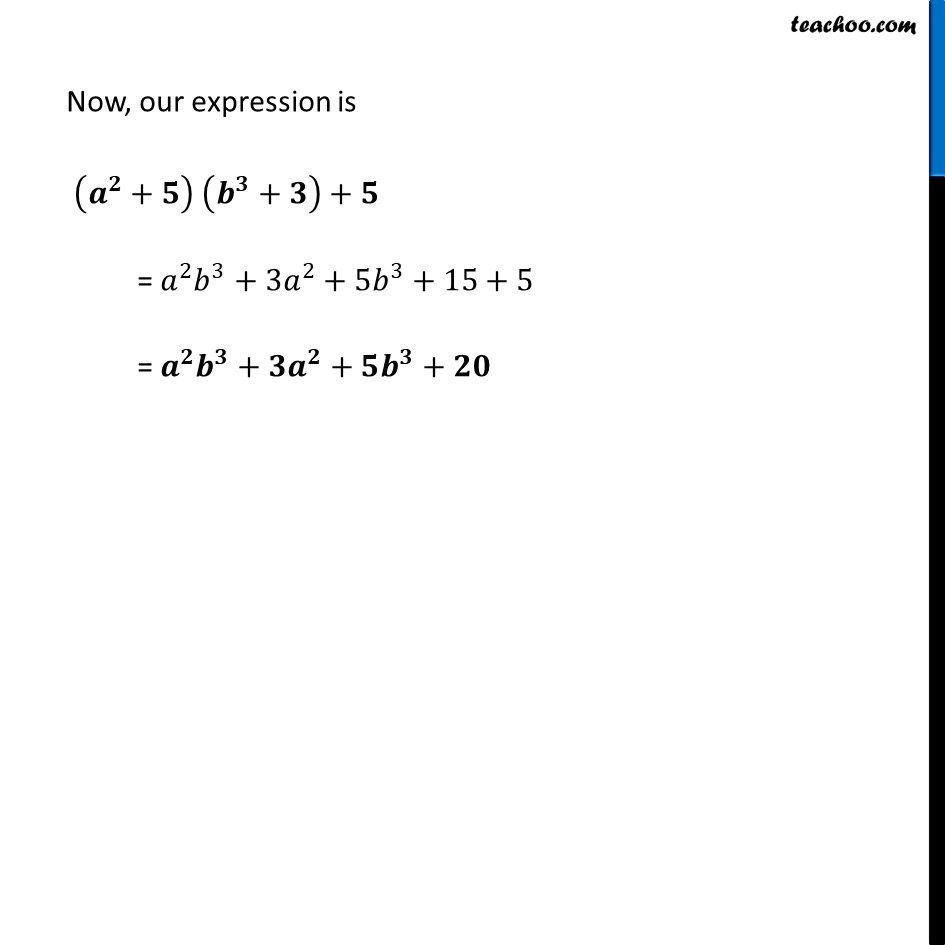Ex 8.4

Chapter 8 Class 8 Algebraic Expressions and Identities
Serial order wiseLearn in your speed, with individual attention - Teachoo Maths 1-on-1 Class

### Transcript

Ex 8.4, 3 Simplify. (ii) (𝑎^2+5) (𝑏^3+3)+5Here, there are 2 expressions: 1st expression = (𝑎^2+5) (𝑏^3+3) 2nd expression = 5 Solving 1st expression (𝒂^𝟐+𝟓) (𝒃^𝟑+𝟑) = 𝑎^2 (𝑏^3+3)+5(𝑏^3+3) = (𝑎^2×𝑏^3 )+(𝑎^2×3)+(5×𝑏^3 )+(5×3) = 𝒂^𝟐 𝒃^𝟑+𝟑𝒂^𝟐+𝟓𝒃^𝟑+𝟏𝟓 Now, our expression is (𝒂^𝟐+𝟓) (𝒃^𝟑+𝟑)+𝟓 = 𝑎^2 𝑏^3+3𝑎^2+5𝑏^3+15+5 = 𝒂^𝟐 𝒃^𝟑+𝟑𝒂^𝟐+𝟓𝒃^𝟑+𝟐𝟎